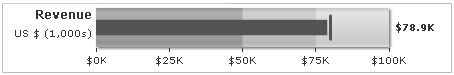You are viewing documentation for an older version. For current documentation - click here.

Here, we'll create a simple bullet graph to show the revenue figures for a fictional company.

The graph will look as under:The various tasks involved in building this graph are:

1. Set chart lower and upper limit.
2. Divide the specified limits into qualitative ranges of performance, such as poor, satisfactory and good.
3. Decide the value to represent.
4. Decide the target figure to represent
5. Choose chart cosmetics.

Let's see how to do each of these.

Data for the graph

First, we decide the qualitative ranges of revenue performance for that company. Let's assume it to something as under:

 Range What it means? Color \$0-\$50,000 Poor Dark gray \$50,000-\$75,000 Moderate Normal gray \$75,000-\$100,000 Good Light gray

Also, we intend to plot the following values:

Intended revenue: \$80,000
Revenue achieved: \$78,900

The XML/JSON for the above data will look as under:

`<chart lowerLimit="0" upperLimit="100" caption="Revenue" subcaption="US \$ (1,000s)" numberPrefix="\$" numberSuffix="K" showValue="1">  <colorRange>    <color minValue="0" maxValue="50" code="A6A6A6"/>    <color minValue="50" maxValue="75" code="CCCCCC"/>    <color minValue="75" maxValue="100" code="E1E1E1"/>  </colorRange>  <value>78.9</value>  <target>80</target></chart>`
```{
"chart": {
"lowerlimit": "0",
"upperlimit": "100",
"caption": "Revenue",
"subcaption": "US \$ (1,000s)",
"numberprefix": "\$",
"numbersuffix": "K",
"showvalue": "1"
},
"colorrange": {
"color": [
{
"minvalue": "0",
"maxvalue": "50",
"code": "A6A6A6"
},
{
"minvalue": "50",
"maxvalue": "75",
"code": "CCCCCC"
},
{
"minvalue": "75",
"maxvalue": "100",
"code": "E1E1E1"
}
]
},
"value": "78.9",
"target": "80"
}```

Here, we've:

• Created the <chart> element, which is the root element of each chart.
• Specified chart limits, caption, sub-caption, number prefix & number suffix. You can specify a lot more properties for the <chart> element, which have been discussed in next sections.
• Defined the color range (numerical value for qualitative ranges) for the chart under the <colorRange> element. Each range value has its own exclusive minValue and maxValue attributes and also a color code.
• Defined the value for chart inside the <value> element.
• Defined the intended target for chart inside the <target> element.